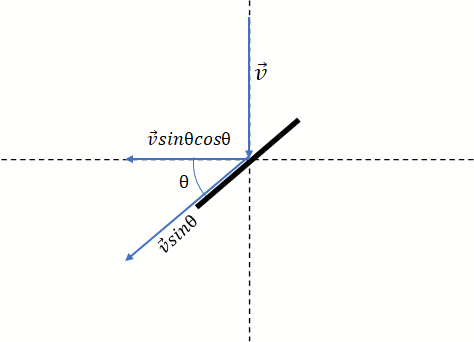# Max linear speed of propeller tip in water

james weaver
Homework Statement:: project not homework
Relevant Equations:: vectors

Hi,

I am trying to determine the maximum linear velocity of a propeller tip when subject to flowing water with velocity ##\vec V##. For simplicity, I will assume that rotational inertial is negligible. The drawing below shows the tip of a propeller blade as viewed directly level from the side. If the following diagram is correct, it implies that the maximum of ##\vec Vsin\theta cos\theta## will occur when ##\theta## is at 45 degrees, and will be equal to exactly ##\frac 1 2\ \vec V##

The relationship seems reasonable as ##\vec Vsin\theta cos\theta## is zero when ##\theta## equals 0 or 90, which is expected.Mentor
Homework Statement:: project not homework
Relevant Equations:: vectors

Hi,

I am trying to determine the maximum linear velocity of a propeller tip when subject to flowing water with velocity ##\vec V##. For simplicity, I will assume that rotational inertial is negligible. The drawing below shows the tip of a propeller blade as viewed directly level from the side. If the following diagram is correct, it implies that the maximum of ##\vec Vsin\theta cos\theta## will occur when ##\theta## is at 45 degrees, and will be equal to exactly ##\frac 1 2\ \vec V##

The relationship seems reasonable as ##\vec Vsin\theta cos\theta## is zero when ##\theta## equals 0 or 90, which is expected.

View attachment 301675
(Thread moved from HH to ME, since it appears to be a general project question).

When you say "max propeller speed", do you mean for a drive propeller which you are trying to avoid cavitation? Or do you mean for an energy conversion turbine that is being driven by the water flow?

james weaver
Energy conversion driven by water flow

Energy conversion driven by water flow
So this is a turbine driven by water, not a propeller moving the water.
There will be twist along the blade. The tip of the blade will trace a helical path in the passing fluid. It is the length of that helix that determines the tip velocity.
Are you assuming that no energy is being extracted from the flow?
What will be the angle of attack of the blade profile when operating?

•berkeman
james weaver
Sorry, I am not very familiar with some of these concepts. For one, I am just assuming that the propeller blades are rectangles that are at a 45 degree angle relative to the axis of rotation of the shaft. Secondly, since I don't have info on the rotational inertia of the shaft, I am trying to determine what RPM would result from this setup (just a rough estimate) given a water velocity of ##\vec V##. My thought process was this: If I want to achieve a given RPM, then what should should the radius of my propeller be? (assuming that the propeller blades were to offer negligible resistance to being rotated).

This is what I have:

##r=\frac {V} {\omega}=\frac { | \vec V sin\theta cos\theta |} {\omega}##

So then ##r## as a function of RPM should be:

##r=\frac { | 30\vec V sin\theta cos\theta |} {RPM\pi}##

Hope this makes sense.

If I want to achieve a given RPM, then what should the radius of my propeller be?
Firstly, stop calling it a propeller if it is actually a turbine, because it is driven by the fluid.
Secondly, inertia is not important, the detailed problems will come when you begin to extract energy from the flow.
Thirdly, from the bulk fluid and energy side of the problem, a propeller or a turbine can be modeled as a "transducer disc", ignoring the internal details.

Simple analysis says for 45° the tip will rotate about the centre at the same speed as the water flow. That means the water will flow along the tip surface at √2 = 1.4142 times the flow velocity.

But the distance around a circle is 2πr, so the shaft will turn once for each 2π radii of fluid that flows through the transducer disc.

james weaver
I'm sorry, I'm confused on how the water could flow across the tip surface at a greater velocity than it's initial velocity.

I'm sorry, I'm confused on how the water could flow across the tip surface at a greater velocity than it's initial velocity.
The length of the tip surface that passes a water molecule is longer than the linear flow down stream, because it is the vector sum of rotation and flow.

james weaver
Ok, I understand the math. I guess I'm just confused then as to what force accelerates the water from ##\vec V## to ##\sqrt2\vec V##. Guess I have something to learn about fluid dynamics.

Guess I have something to learn about fluid dynamics.
We are all in that position, always.

The water moves at a fixed speed. The blade rotates at a fixed speed. The contact between the turbine blade and the water is in shear. The differential velocity across that laminar flow is greater than the linear flow of the water downstream. The wetted area surface drag is dependent on blade angle.

Gold Member
Easy version:

Since the blade is rotating, it moves at an angle (depending on its pitch) to the water flow.

The result is you have the water flowing thru the turbine at some speed and the blade tip moving across the water flow. These two add by vector addition.

Since you chose a blade angle of 45°, you can think in terms of a square with one side being the water flow speed and an adjacent side being the blade tip rotational speed. If you draw a diagonal in the square, the diagonals length is the vector sum of the two speeds.

That's why the tip speed thru the water is faster than the straight-line water flow rate.

Hope this helps!

Cheers,
Tom

Last edited:
james weaver
Thanks guys for these very helpful responses.

•berkeman and Tom.G